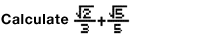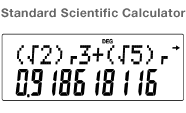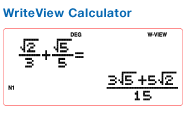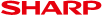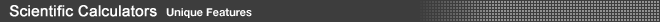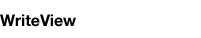A standard scientific calculator cannot display fractions and symbols such as “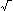” the way they appear in a proper mathematical formula. A WriteView calculator, however, can display fractions and symbols just as they appear in a mathematical formula.